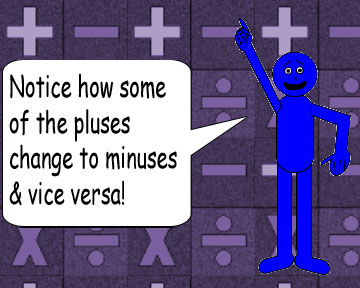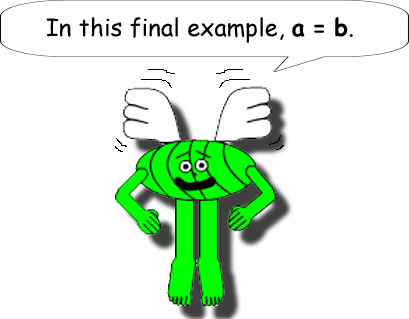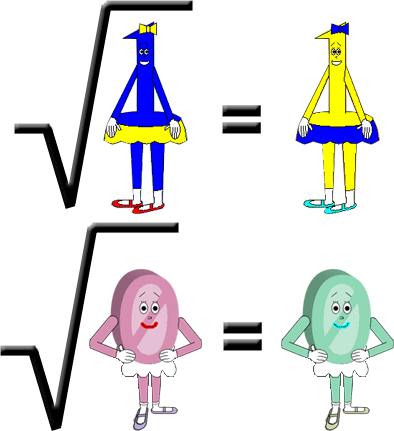# Cunning Cosine!

## Examples:

√(32 + 42 - 2 • 12cos(360°)) = √(9 + 16 - 24cos(360°)) = √(25 - 24) = 1

(A = 3 & B = 4 in this 1st example; their product is 12; |3 - 4| = 1)

√(152 + 112 - 2 • 165cos(360°)) = √(225 + 121 - 330cos(360°)) = √(346 - 330) = √(16) = 4

(A = 15 & B = 11 in this 2nd example; their product is 165; |15 - 11| = 4)

√(132 + 82 - 2 • 104cos(360°)) = √(169 + 64 - 208cos(360°)) = √(233 - 208) = √(25) = 5

(A = 13 & B = 8 in this 3rd example; their product is 104; |13 - 8| = 5)

### If the variables A & B have different signs, the minus sign in the formula turns into a plus sign because the product of the 2 variables will be negative. This number then gets multipied by 2. (Positive 2) Remember that subtracting negative numbers is the same as adding their positive additive inverses!

√(22 + (-6)2 - 2 • -12cos(360°)) = √(4 + 36 + 24cos(360°)) = √(40 + 24) = √(64) = 8

(A = 2 & B = -6 in this 4th example; their product is -12; |2 - (-6)| = |2 + 6| = 8)

√((-2)2 + (-6)2 - 2 • 12cos(360°)) = √(4 + 36 - 24cos(360°)) = √(40 - 24) = √(16) = 4

(A = -2 & B = -6 in this 5th example; their product is +12; |-2 - (-6)| = |-2 + 6| = 4)

√((-2)2 + 62 - 2 • -12cos(360°)) = √(4 + 36 + 24cos(360°)) = √(40 + 24) = √(64) = 8

(A = -2 & B = 6 in this 6th example; their product is -12; |-2 - 6| = |-8| = 8)### I'm sure that you already know that squaring negative numbers gives you a positive number instead of a negative number! It's because of how multiplication works for negative numbers. Please see the Web page entitled: "What Type of Number Will You Get?" for more details. Also, know that negative numbers have imaginary square roots.Note: If you plug imaginary or complex numbers into the variables A or B, then the result in the formula will sometimes be a complex number that matches their difference in absolute value!(The variables aren't capitalized in Brain's word balloon, but let's not be case-sensitive!)

√(52 + 52 - 2 • 25cos(360°)) = √(25 + 25 - 50cos(360°)) = √(50 - 50) = 0

(A = 5 & B = 5 in this 7th & final example; their product is 25; |5 - 5| = 0)

It's that simple! When A = B, the result in the formula is zero(0) since any number minus itself is always zero!

## And remember this fact about the 2 idempotent numbers 1 & 0: